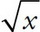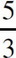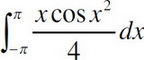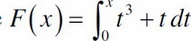# AP Calculus AB Practice Test 19

### Test Information8 questions24 minutes

Calculator Allowed

1. If f(x) = x-3 + 3+ 5π - e2, then f′(x) =

2. Find the absolute maximum of y =x3 - x2 - 7x on the interval [-2, 2].

3. Approximate the area under the curve y = x2 + 2 from x = 1 to x = 2 using four right-endpoint rectangles.

4. Approximate the area under the curve y = x2 + 2 from x = 1 to x = 2 using four inscribed trapezoids.

5. Evaluate.

6. Suppose. What is the change in f(x) as t increases from 1 to 4.

7. In the xy-plane, 2x + y = k is tangent to the graph of y = 2x2 - 8x + 14. What is the value of k ?

8. The function f is continuous on the closed interval [0,4] and twice differentiable over the open interval, (0, 4). If f′(x) < 0 and f″(x) > 0 over the interval (0, 4), which of the following could be a table of values for f ?